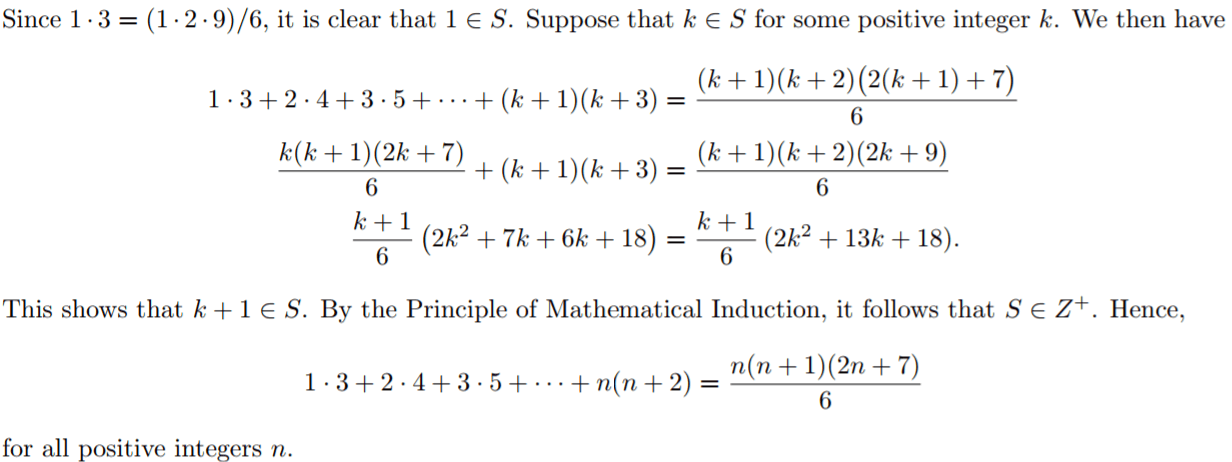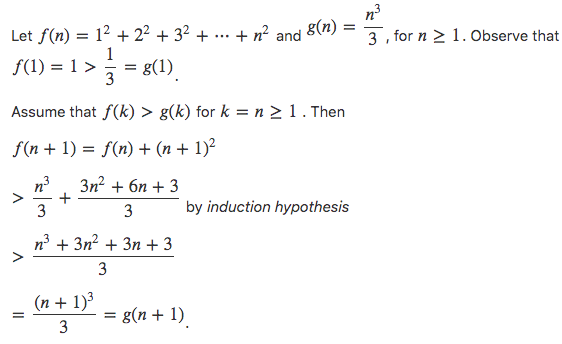# RBSE Maths Class 11 Chapter 4: Important Questions and Solutions

RBSE Maths Chapter 4 – Principle of mathematical induction Class 11 Important questions and solutions is available here. The important questions and solutions of Chapter 4, available at BYJU’S, contain detailed explanations for the better understanding of the students. All these important questions are based on the new pattern prescribed by the RBSE. Students can also get the syllabus and textbooks on RBSE Class 11 solutions.

Chapter 4 of the RBSE Class 11 Maths will help the students to solve problems related to the principle of mathematical induction.

### RBSE Maths Chapter 4: Exercise 4.1 Textbook Important Questions and Solutions

Question 1: If statement is P(n) : (n + 3) < 2n+3 then write P(4).

Solution:

P(n) : (n + 3) < 2n+3

Putting n = 4

P(4) : (4 + 3) < 24+3

P(4) : 7 < 27

P(4) : 7 < 128 is true

Hence, P(4) : 7 < 27

Question 2: Write the nth term of 1 + (1 + 3) + (1 + 3 + 5) + …….

Solution:

1 + (1 + 3) + (1 + 3 + 5)+…….

= 1 + (1 + 3) + (1 + 3 + 5) + ……. + {1 + 3 + 5 + … + (2n – 1)}

Tn = a + (n – 1) d

= 1 + (n – 1) 2

= 1 + 2n – 2

Tn = 2n – 1

Hence, nth term = 1 + 3 + 5 + … + (2n – 1).

Question 3: Write nth term of 1.4.7 + 2.5.8 + 3.6.9 + …….

Solution:

nth term of 1.4.7 + 2.5.8 + 3.6.9 + …….

= (nth term of 1, 2, 3, …) . (nth term of 4, 5, 6, …) . (nth term of 7, 8, 9, …)

= [1 + (n – 1) – 1] . [4 + (n – 1) – 1] . [7 + (n – 1)1]

= (1 + n – 1) . (4 + n – 1) . (7 + n – 1)

= n (n + 3) . (n + 6)

Hence, nth term = n . (n + 3) . (n + 6)

Question 4: Prove that 1 + 3 + …… + (2n – 1) = n2 .

Solution:

Let the given statement is P(n) where n ∈ N

P(n) : 1 + 3 + … + (2n – 1) = n2 when n = 1, P(1) = 1 = 12 = 1

Hence, the given statement is true for n = 1.

So, P(1) is true Let given statement is true for n = m that is,

P(m) = 1 + 3 + … + (2m – 1) = m2 …(i)

Now, to prove that given the statement is true for n = m + 1,

P (m + 1) is true.

Now, P(m + 1) = 1 + 3 + …… + {2(m + 1) – 1} = (m + 1)2 = 1 + 3 + … + ( – 1) = (m + 1)2

Hence, the given statement is true for n = m + 1,

P (m + 1) is true.

Hence, from the principle of mathematical induction, the given statement is true for each natural number n ∈ N.

Question 5: Prove that 1 + 4 + 7 + . . . + (3n − 2) = [n * (3n − 1)] / 2.

Solution:

We prove by induction that

Sn : 1 + 4 + 7 + . . . + (3n − 2) = [n * (3n − 1)] /  is true for all natural numbers n.

The statement S1 : 1 = [1(3 − 1)] /  is true.

Assume that Sk : 1 + 4 + 7 + . . . + (3k − 2) = [k (3k − 1)] /  is true and prove that

Sk+1 : 1 + 4 + 7 + . . . + (3(k + 1) − 2) = [(k + 1) (3 (k + 1) − 1)] /  is true.

Observe that

1 + 4 + 7 + . . . + (3 (k + 1) − 2) = (1 + 4 + 7 + . . . + (3n − 2)) + (3k + 1)

= [k (3k − 1)] /  + (3k + 1)

= [k (3k − 1) + 2 (3k + 1)] / 

= [3k2 − k + 6k + 2] / 

= [3k2 + 3k + 2k + 2] / 

= [(k + 1) (3k + 2)] / 2

= [(k + 1) (3 (k + 1) − 1)] / 

Thus, by the Principle of Math Induction Sn is true for all natural numbers n.

Question 6: Prove that 1.3 + 2.4 + ……. + n (n + 2) = [n * (n + 1) * (2n + 7)] / .

Solution:

We will use the Principle of Mathematical Induction. Let S be the set of all positive integers n such that

1.3 + 2.4 + ……. + n (n + 2) = [n * (n + 1) * (2n + 7)] / .Question 7: Prove that 1 + 5 + 52 + …….. 5n-1 = [5n – 1 / 4].

Solution:

P (n) = 1 + 5 + 52 + …….. 5n-1 = [5n – 1 / 4]

For n = 1,

P (1) = (51 – 1 / 4) = 4 / 4 = 1

P (1) is true.

For n = m,

P (m) = 1 + 5 + 52 + …….. 5m-1 + 5m = [5m – 1 / 4] —– (1)

To prove that the statement is true for n = m + 1

P (m + 1) = 1 + 5 + 52 + …….. 5m-1 + 5m

= [5m – 1 / 4] + 5m

= [5m – 1 / 4 * 5m] / 

= [5m+1 – 1] / 

Hence, P(m + 1) is also true. So, by the principle of mathematical induction, the given statement is true for each natural number n ∈ N.

Question 8: Solve by the principle of mathematical induction, 2n > n.

Solution:

Let P(n) = 2n > n

When n = 1, 21 > 1

Hence, P(1) is true.

Let P (m) be true for n = m

P (m) : 2m > m ……(i)

Now, it has to be proved that the given statement is also true for n = m + 1 that is P(m + 1) has to be true.

Multiplying by 2 on both sides of equation (i), we have

2 * 2m > 2m

⇒ 2m+1 > 2m = m + m > m + 1

Thus, P (m + 1) is true.

Hence, by the principle of mathematical induction, P(n) is true for each natural number n ∈ N.

Question 9: Solve by the principle of mathematical induction, (1 + x)n ≥ 1 + nx, x > 0

Solution:

Let P(n) : (1 + x)n ≥ (1 + nx), x > 0

When n = 1 then P(n) is true

⇒ (1 + x) ≥ (1 + x) is true for x > -1 …(i)

For n = m

P (m) : (1 + x)m ≥ (1 + mx), x > 0 is true …(ii)

Now, we have to prove that P(m + 1) is true.

(1 + x)m+1 = (1 + x)m (1 + x)

Given x > 0.

So, (1 + x) > 0

Hence, using (1 + x)m ≥ (1 + mx) we get

(1 + x)m+1 ≥ (1 + mx) (1 + x)

(1 + x)m+1 ≥ (1 + x + mx + mx2) …..(iii)

Since, m is a natural number and x2 ≥ 0 is such that mx2 ≥ 0

So, (1 + x + mx + mx2) ≥ (1 + x + mx)

⇒ (1 + x)m+1 ≥ (1 + x + mx)

⇒ (1 + x)m+1 ≥ [1 + (1 + m)x]

The statement (ii) is true.

Hence, by the principle of mathematical induction P(n) is true for each natural number n ∈ N.

Question 10: Prove that 23n – 1 is divisible by 7.

Solution:

P(n) : 23n – 1 is divisible by 7 that is 23n – 1 = 7n

For n = 1, P(1) = 23(1) – 1 = 7 is divisible by 7

Hence, given statement is true for n = 1, i.e., P(1) is true.

Let P (m) is true, that is 23m – 1 = 7m ……(i)

Now, to prove that the given statement is also true for n = m + 1 i.e.. P(m + 1) is true that is 23(m+1) – 1

= 23m+3 – 1

= 23m * 23 – 1

= (7m + 1)* 23 – 1

= 56m + 8 – 1

= 7 (8m + 1)

23(m+1) – 1 is divisible by 7.

⇒ P(m + 1) is true.

Hence, the given statement P(n) is true for each value of n.

Question 11: Prove that: 10n + 3.4n+2 + 5, is divisible by 9.

Solution:

Let the given statement 10n + 3.4n+2 + 5 be divisible by 9.

For n = 1,

P(1) = 101 + 3.41+2 + 5

= 10 + 3 * 43 + 5

= 10 + 192 + 5

= 207 is divisible by 9.

Hence, the given statement is true for n = 1.

P(1) is true.

Let the statement P(m) be true, that is

P(m) = 10m + 3.4m+2 + 5 is divisible by 9.

Let 10m + 3 * 4m+2 + 5 = 9m

Now, to prove that the given statement is true for m + 1, that is P(m + 1) is true.

P(m + 1) = 10m+1 + 3 * 4m-1+2 + 5

= 10m * 1010 + 3 * 4m+2 * 4 + 5

= (9m – 3 * 4m+2 – 5) * 10 + 3 * 4m+2 * 4 + 5

= 9 * [10m – 2 * 4m+2 – 5] is divisible by 9.

Hence, the given statement is true for n = m + i, i.e., P(m + 1) is true.

Hence, P(n) is true for each natural number n ∈ N.

Question 12: Prove that 41n – 14n is divisible by 27.

Solution:

Let the given statement is P(n) where n ∈ N, that is P(n) = 41n – 14n is divisible by 27

For n = 1,

P(1) = 411 – 141

= 41 – 14

= 27 which is divisible by 27

Hence, the given statement is true for n = 1, i.e., P( 1) is true

Let given statement is true for n = m that is P(m) is true.

Then P(m) = 41m – 14m is divisible by 27 ……(i)

Now, to prove that the given statement is true for n = m + 1,

P(m + 1) is also true.

Now,

P(m + 1) = 41m+1 – 14m+1

= 41m+1 – 41 * 14m + 41 * 14m – 14m+1

= 41 (41m – 14m) + 27 * (14m)

Here, there are two terms in R.H.S. The first term (41m – 14m) is divisible by 27 [from (i)] and the second term is also divisible by 27 because 27 is its one factor. Then the sum of both terms is divisible by 27. Hence, the principle of mathematical induction, the given statement is true for each natural number n ∈ N.

Question 13: Prove that 12 + 22 + …… + n2 > n3 / 3.

Solution:Thus, f (n + 1) is true, when f (n) is true.

Hence, by the principle of mathematical induction, f (n) is true for n ∈ N.